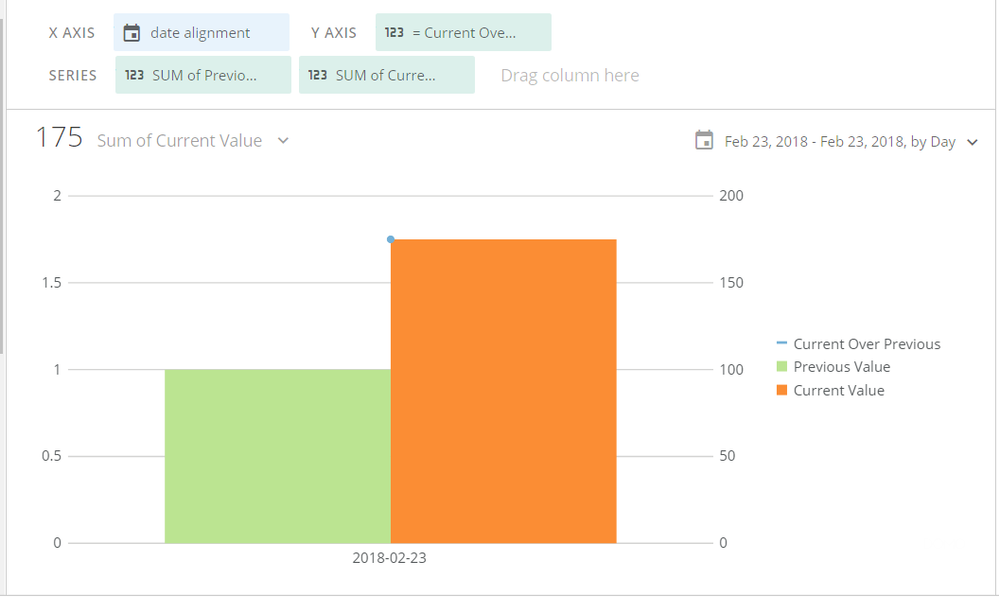# Secondary Y Axis

Wondering if there's a way to create a secondary Y Axis? In Excel you can do this on a combo chart in order to show multiple metrics on one graph. Additionally is the only way to layer a line over bar with the YoY graphs?

• Hey @user02428,

Out of the box Domo will allow you to combine metrics with different types on the same graph. YFrom your example, you could put both total impressions and average CTR metrics on a grouped bar and line or multi line graph and Domo will create a secondary axis based on the datatypes of the different metrics. The first series listed will be the left axis and the second metric will be the right axis.

Screenshot below:Let me know if this is helpful.

**Say 'Thanks' by clicking the thumbs up in the post that helped you.

• Please take a look at the following post/solution and let me know if this answers your question:

https://dojo.domo.com/t5/Card-Building/Is-there-a-way-to-replicate-an-Excel-combo-chart-in-Domo/m-p/6049

• So that solution would allow you to only show one metric across the time and broken up by the series. I'm wondering if there's a way to show multiple metrics such as sum of impressions and average CTR? This would require a secondary axis because they would have two different calculations and would be two different number types.

• Hey @user02428,

Out of the box Domo will allow you to combine metrics with different types on the same graph. YFrom your example, you could put both total impressions and average CTR metrics on a grouped bar and line or multi line graph and Domo will create a secondary axis based on the datatypes of the different metrics. The first series listed will be the left axis and the second metric will be the right axis.

Screenshot below:Let me know if this is helpful.

**Say 'Thanks' by clicking the thumbs up in the post that helped you.

• @user02428 wrote:

So that solution would allow you to only show one metric across the time and broken up by the series. I'm wondering if there's a way to show multiple metrics such as sum of impressions and average CTR? This would require a secondary axis because they would have two different calculations and would be two different number types.

Was this resolved? I'm curious and trying to do this as well.

Thank you!

• I agree - I don't think this answers my question.

I have the following data:

week_beginning, active_people_this_week (1),active_people_last_4_weeks (2), =(1)/(2) (calculated filed)

I want (1) & (2) to be on the left y axis, and the =(1)/(2) (calculated filed) to be on the right axis. How do I do this step by step?

Thanks!

• What Domo is doing (attached)

This discussion has been closed.